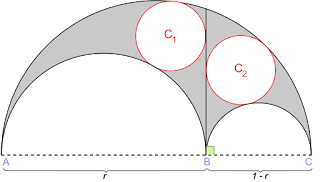## On Fractals of the ArbelosArbelos Sculpture in Netherlands

I wrote recently about the Arbelos, but mostly about its history. A problem posed there led to a discussion with a heady young man who had a method I had never seen before(Ok, I still haven't seen it, but his overview told me I didn't know that one. So in this post, I want to provide what I think is the common proof, and then point out some other facts about the arbelos proceeding into the explanation (I hope) of the cryptic use of fractal in the title.

First the Proof that the area of the arbelos itself (the shape cut out by the two smaller semi-circles from the larger) is proportional to the square of the length of the segment from the common point on the two small circles to the intersection F on the larger circle.

If you draw the triangle AFB, it is a right triangle (inscribed in a semi-circle) and so the length of the segment AF squared is equal to the product of the two diameters of the smaller semi-circles.  (Remember that triangle, BTW, it will be mentioned again.

Now reflect the whole image over the x-axis, and you get three circles.  The arbelos is clearly the area of the larger circle minus the two smaller ones.  Working with diameters for a minute, let's call the diameters of the two smaller circles D1 and D2, and the larger one just D.  The Diameter of the larger circle is  D1 + D2.

So the Areas of the two copies of the Arbelos is given by (D1 + D2)2 $\frac{\pi}{4}$  = (D12 + D2 2 + 2D1 D2 )  $\frac{\pi}{4}$.  Subtracting the area of the two smaller circles leaves us with 2D1 D2   $\frac{\pi}{4}$  . The arbelos is half this, so we have that CF $\frac{\pi}{4}$= D1 D2$\frac{\pi}{4}$

Now on to some fun stuff.

Oh, but wait! You remember the right triangle AFB?  The two points where it crosses the two smaller semi-circles, will connect to form a line tangent to both of them.  And the two tangent points, and the endpoints of CF form a rectangle. And of course that rectangle is inscribable inside a circle, and that circles area is twice the area of the arbelos.

So one of the things the arbelos is famous for is the circles of Archimedes, two circles in the arbelos that are each tangent to the line CF, one small semi-circle, and the large semi-circle.*wik
Those semi-circles have diameters which are the square root of the arbelos.  And if you fit the smallest circle that inscribes them both, it has the same area as the arbelos.

If you take a moment to measure the three semi-circular arc lengths, you find that the sum of the two smaller is the same as the larger.  You can prove this is always true by using a unit diameter circle, and if it's true there, it's true on any scaler multiplication of the figure.  On the unit diameter semi-circle the arc length is $\frac{ \pi}{2}$ .  Calling the two smaller circles d and 1-d , we see that we get the same result.

The stage two Fractal arbelos
But wait, there's more.   Do that again!  Break each of the two smaller semi-circles down into two smaller semi-circles in the same proportion as D1 : D2

The sum of all four of the smallest arc lengths is still equal to the arc length of the largest one.  And since each small arbelos is a scaler reduction of the original, we can find that their area by simply applying the scale factor.  So the area of the one on the left is    $\frac{D_1}{D_1+D_2}$* original arbelos.  The one on the right similarly would be  $\frac{D_1}{D_1+D_2}$* original arbelos.  Oh, and the two in the middle of the four....... congruent... (nah, you didn't see that coming!).

Well that's probably plenty for one post, some people have papers to grade.  Be well.

#### 1 comment:

TLexen said...

I have an interest in symmetrical arbeli (with the two minor arcs the same), and in truncated symmetrical arbeli (where the two minor arcs are the same, but less than 180 degrees; and the minor and major arcs have the same arc angle; and the arc ends are in line). Looking for any info or resources.